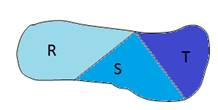Chapter 8.1, Problem 44EElementary Geometry For College St...

7th Edition
Alexander + 2 others
ISBN: 9781337614085

Solutions

Chapter
SectionElementary Geometry For College St...

7th Edition
Alexander + 2 others
ISBN: 9781337614085
Textbook Problem

Given region R ∪ S ∪ T , explain why A R ∪ S ∪ T > A R + A S + A T .To determine

To explain:

ARST=AR+AS+AT

If region RST is given byExplanation

Given,

From the figure, region R, S, and T are mutually exclusive and exhaustive.

(i.e.) RST

Where RST=R+S+T

Area of the region R, S, and T is AR,AS, and AT.

Thus, the area of the figure is ARST

(i

Still sussing out bartleby?

Check out a sample textbook solution.

See a sample solution

The Solution to Your Study Problems

Bartleby provides explanations to thousands of textbook problems written by our experts, many with advanced degrees!

Get Started

1. Maximize subject to the constraints determined by the shaded region shown in the figure.

Mathematical Applications for the Management, Life, and Social Sciences

Calculate y'. 4. y=tanx1+cosx

Calculus: Early Transcendentals

In Exercises 4148, find the indicated limit given that limxaf(x)=3 and limxag(x)=4 41. limxa[f(x)g(x)]

Applied Calculus for the Managerial, Life, and Social Sciences: A Brief Approach

Write each expression in terms of i. 18

Trigonometry (MindTap Course List)

True or False: div curl F = 0.

Study Guide for Stewart's Multivariable Calculus, 8th

The horizontal asymptote(s) for f(x)=|x|x+1 is (are): a) y =0 b) y = 1 c) y = 1 d) y = 1 and y = 1

Study Guide for Stewart's Single Variable Calculus: Early Transcendentals, 8th